## Electrostatics Cheatsheet

Electrostatics Cheat Sheet

Structure of atom:

Positively Charged Particles

In this type of particles, numbers of positive ions are larger than the numbers of negative ions. In other words numbers of protons are larger than the number of electrons.

p+>e-

Negatively Charged Particles

In this type of particles, numbers of negative ions are larger than the numbers of positive ions. In other words numbers of electrons are larger than the number of protons.

e+>p-

Neutral Particles

These types of particles include equal numbers of protons and electrons. Be careful, they have both protons, neutrons and electrons however, numbers of “+” ions are equal to the numbers of “-” ions.

e+=p-

Atoms having same charge repel each other and atoms having opposite charges attract each other.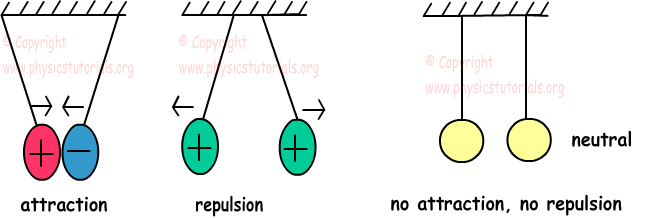Types of Charging

Charging means gaining or losing electron. Matters can be charged with three ways, charging by friction, charging by contact and charging by induction.

Charging by Friction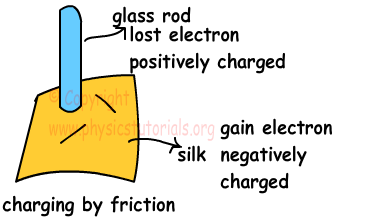When you rub one material to another, they are charged by friction. Material losing electron is positively charged and material gaining electron is negatively charged. Amount of gained and lost electron is equal to each other.

Charging by Contact

There are equal numbers of electrons and protons in a neutral matter. If something changes this balance we can say it is charged.

1. When charged object touches to a neutral object, they both have same charge.

2. When two charged matter touch each other, total charge of the system is conserved and they share the total charge according to their capacities. If they have same amount of different charges, when we touch one another they become neutral. If the amount of charges is different then, after flow of charge they are both negatively or positively charged. Having opposite charges after contact is impossible.

Charging by Induction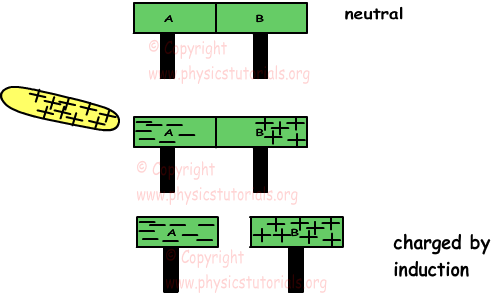A and B conductors are neutral at the beginning. When we put a positively charged plate near them, it attracts the electrons in the conductors. Electrons move to the left part and protons stays. Thus, when we separate plates A and B they are charged by induction, A is negatively charged and B is negatively charged. Be careful, there is no contact; they are charged only by induction.

Electroscope

It is a device that is used for detecting whether an object is charged or uncharged. It is also determine the type of charge.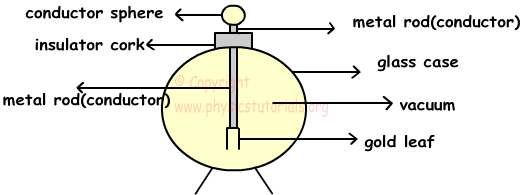Electrical Forces Coulomb’s Law

Coulomb made some experiments and find following equation of electrical forces.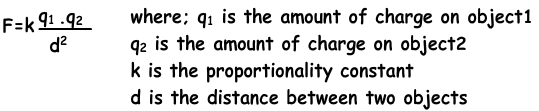· If the objects have same type of charge then the force is repulsive, if they have opposite charges then force is attractive.

· Repulsive or attractive electrical forces is equal in magnitude but opposite in direction, it does not depend on the magnitudes of charges.

F1=-F2

Electric Field

A charged particle exerts a force on particles around it. We can call the influence of this force on surroundings as electric field.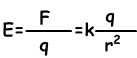Electric field is a vector quantity. And it decreases with the increasing distance. k =9.109N.m2/C2

Force Acting on a Charged Particle inside Electric Field

E=F/q

F=E.q where; F is the force acting on the charge inside the electric field E.Using this equation we can say that;

If q is positive then F=+E.q and directions of Force and Electric Field are same

If q is negative then F=-E.q and directions of Force and Electric Field are opposite.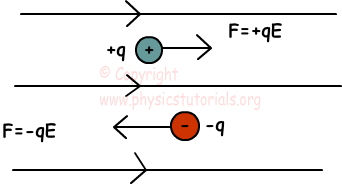Electric Potential and Electric Potential Energy

We learned that in work power energy chapter, objects have potential energy because of their positions. In this case charge in an electric field has also potential energy because of its positions.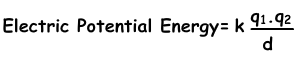Electric Potential

Electric potential is the electric potential energy per unit charge. It is known as voltage in general, represented by V and has unit volt (joule/C).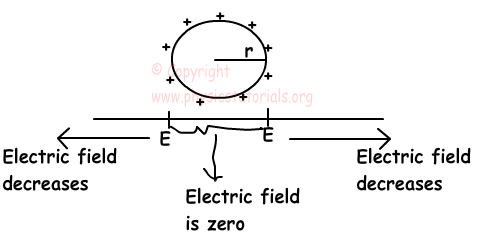V=k.q/d

V is a scalar quantity. If q is negative then V becomes negative, or if q is positive then V becomes positive.

Surfaces having equal potentials are called equipotential surfaces.

Capacitance and Capacitors

Capacitance is the ratio of charged gained per potential gained of the conductors. Unit of capacitance is Coulomb per Volt and it is called as Farad (F).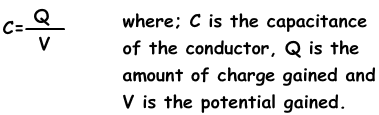Capacitance is a scalar quantity.

Capacitors

Capacitors are devices designed for storing charge. They are commonly used in computers or electronic systems.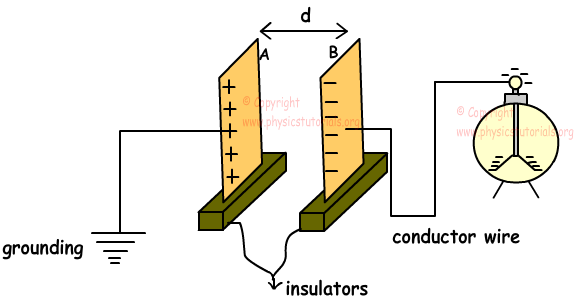The capacitance of the plates is found with the following formula;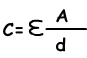Capacitors in Series and Parallel

In this circuit capacitors are connected in parallel.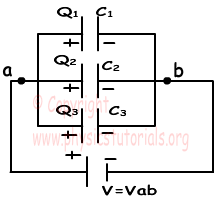We find the charge of each capacitor as;

Q1=C1.V

Q2=C2.V

Q3=C3.V

Ceq=C1+C2+C3

Now we will see the capacitors in series.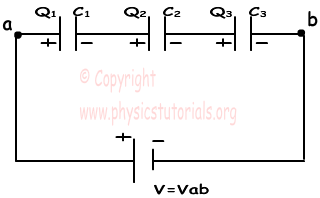Each capacitor has same charge with battery.

C1.V1=Q

C2.V2=Q , V=V1+V2+V3 and Q=Ceq.V

C3.V3=Q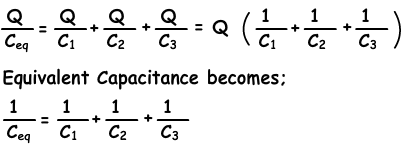Electrostatics Exams and Solutions

Author: